## BBC Micro:bit

The BBC Micro:bit is a low-cost controller board half the size of a credit card, pioneered by the BBC in the UK. The latest version is Version 2.

### Installing uLisp on the BBC Micro:bit

You can install the ARM Version uLisp on a BBC Micro:bit using the Arduino IDE as follows:

• In the Arduino IDE open the Preferences dialog box, and add the following URL to Additional Board Manager URLs:
`https://sandeepmistry.github.io/arduino-nRF5/package_nRF5_boards_index.json`
• Open the Boards Manager… from the Board item on the Tools menu, search for nRF5, and install Nordic Semiconductor nRF5 Boards by Sandeep Mistry.
• Connect the BBC Micro:bit to your computer using a USB cable.
• From the Board menu select BBC micro:bit for version 1.3 or 1.5, or BBC micro:bit V2 for version 2.
• Set Softdevice to None, and set Port to the USB port.

You should now be able to upload uLisp to the BBC Micro:bit.

Follow the instructions in Using uLisp to interact with uLisp on the BBC Micro:bit.

#### Updating the serial buffer

As of uLisp Version 3.6 there is no longer a problem pasting large Lisp programs in via the Serial Monitor, so there is no need to change the serial buffer size from the default.

### BBC Micro:bit V2

The BBC Micro:bit V2 is based on a Nordic Semiconductor nRF52833 ARM Cortex-M4 microcontroller, running at 64 MHz, and it provides 512 Kbytes of flash program memory, and 128 Kbytes of RAM: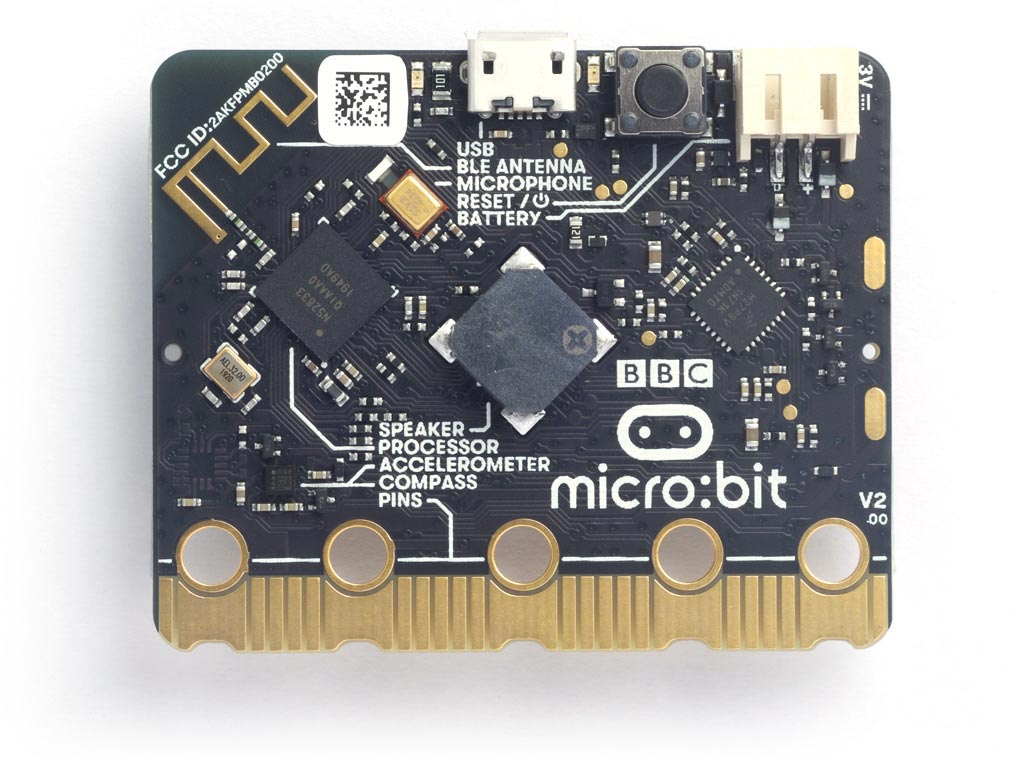It supports the ARM Assembler; see ARM assembler overview.

#### Pin connections

The BBC Micro:bit provides 19 digital input/output pins, 6 analogue inputs, and 19 analogue outputs. They are accessible from the edge connector as follows: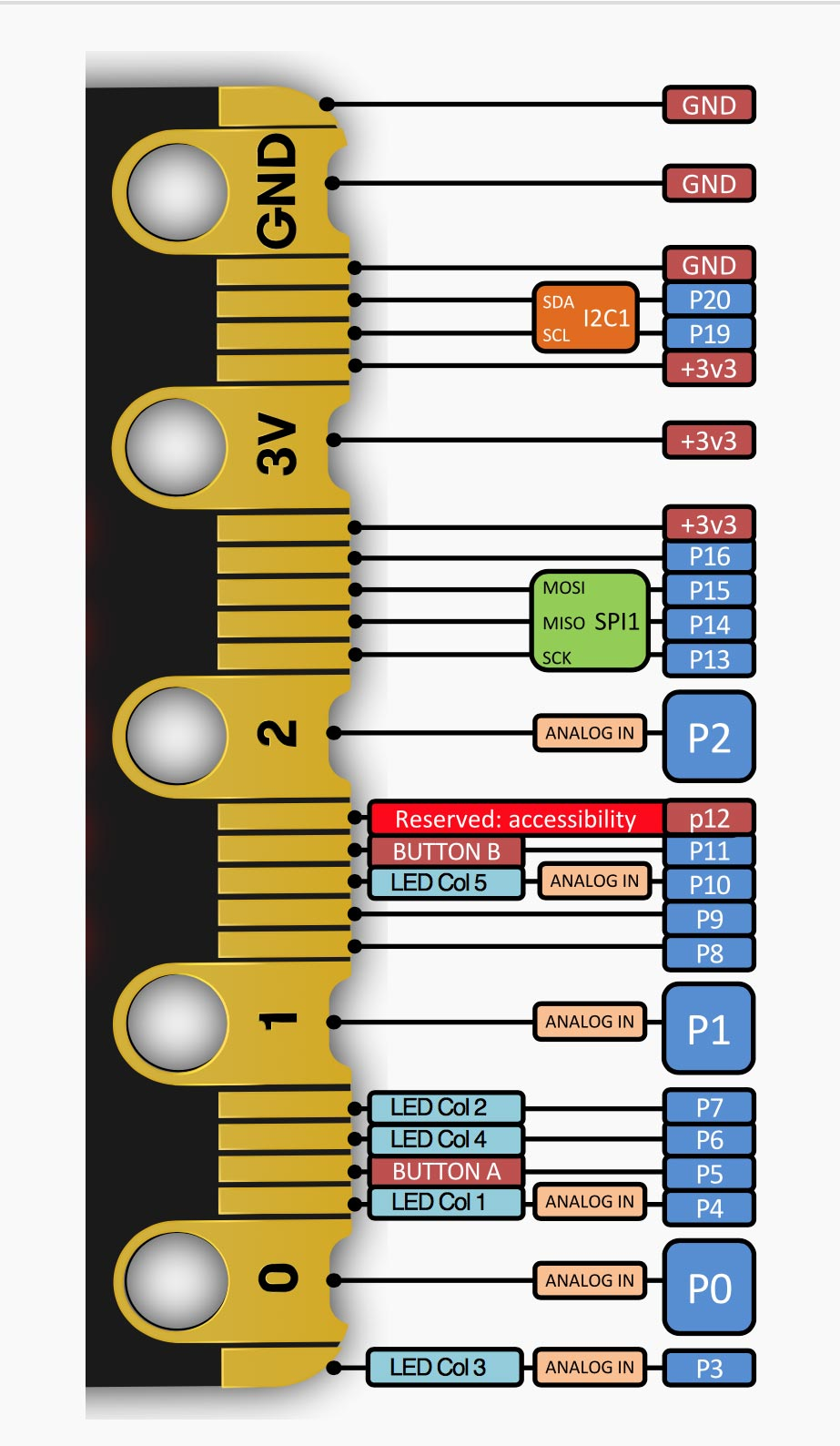For more detailed information about the edge connector see the microbit.org site . The board also includes two pushbuttons and a matrix of 5 x 5 multiplexed LEDs. See Example programs below for examples of using these from uLisp.

#### Buttons

The reverse side of the BBC Micro:bit provides two pushbuttons on pins 5 and 11. You can read these with the following program:

```(defun buttons ()
(pinmode 5 :input)
(pinmode 11 :input)
(loop
(print
(delay 1000)))```

Run it by typing:

`(buttons)`

#### LEDs

The reverse of the BBC Micro:bit also provides a matrix of 5 x 5 LEDs. In the BBC Micro:bit Version 2 these are connected in a more logical matrix of five rows and five columns, so to light any individual LED you need to take the column low and the row high corresponding to that LED.

The following table shows the pin numbers for the rows and columns:

 4 7 3 6 10 21 • • • • • 22 • • • • • 23 • • • • • 24 • • • • • 25 • • • • •

This program flashes the LED at (row, column):

```(defun blink (row column)
(let (x)
(pinmode row :output)
(pinmode column :output)    (digitalwrite row t)
(loop
(digitalwrite column x)
(delay 1000)
(setq x (not x)))))```

For example, to flash the centre LED run:

`(blink 23 3)`

#### Analogue outputs

Any pin can also be used as an 8-bit analogue output, so you can pulsate the LED at (row, column) slowly on and off with the following program:

```(defun pulse (row column)
(let (down)
(pinmode row :output)
(digitalwrite row t)
(loop
(dotimes (x 256)
(delay 5)
(analogwrite column (if down (- 255 x) x)))
(setq down (not down)))))```

Run it by typing:

`(pulse 23 3)`

Exit from any program by entering ~.

#### Analogue inputs

Like the Version 1, the BBC Micro:bit Version 2 provides seven 10-bit analogue inputs, three of which are available on the edge connector pins labelled 0, 1, and 2. The following example program reads the analogue input on pin 0 and displays the value as a bar of LEDs, using the fourth row of LEDs on the matrix.

Here's the program:

```(defun meter ()
(let ((col '(4 7 3 6 10))
(row 24))
(pinmode row :output)
(digitalwrite row t)
(mapc (lambda (x) (pinmode x :output)) col)
(loop
(dotimes (n 5)
(digitalwrite
(nth n col)
(<
(* (1+ n) 170)))))))```

To run it evaluate:

`(meter)`

If you touch pin 0 on the edge connector you'll see the bar flash from the voltage on your finger. Connect a voltage of up to 3.3V to pin 0 to display a bar of LEDs in proportion to the voltage.

#### I2C ports

The BBC Micro:bit V2 provides two I2C ports. Port 0 is connected to the edge connector, and Port 1 provides access to the internal I2C devices.

To access the internal I2C devices give the with-i2c command with the port as a second parameter. For example, to scan port 1 give:

```(defun scn ()
(dotimes (p 127)
(with-i2c (str 1 p)
(when str (print p)))))```

This displays the following device addresses:

 Address Device 25 (#x19) LSM303AGR accelerometer 30 (#x1E) LSM303AGR magnetometer 112-114 (#x70-#x72) NXP KL27Z interface processor

#### LSM303AGR accelerometer/magnetometer

The LSM303AGR sensor contains both an accelerometer and a magnetometer. For details of using it see:

LSM303D Accelerometer Magnetometer

### Original BBC Micro:bit

The original BBC Micro:bit is based on a Nordic Semiconductor nRF51822-QFAA ARM Cortex-M0 microcontroller, running at 16 MHz, and it provides 256 Kbytes of flash program memory and 16 Kbytes of RAM. Version 1.5 is similar except that it has a single LSM303AGR sensor which contains both an accelerometer and a magnetometer.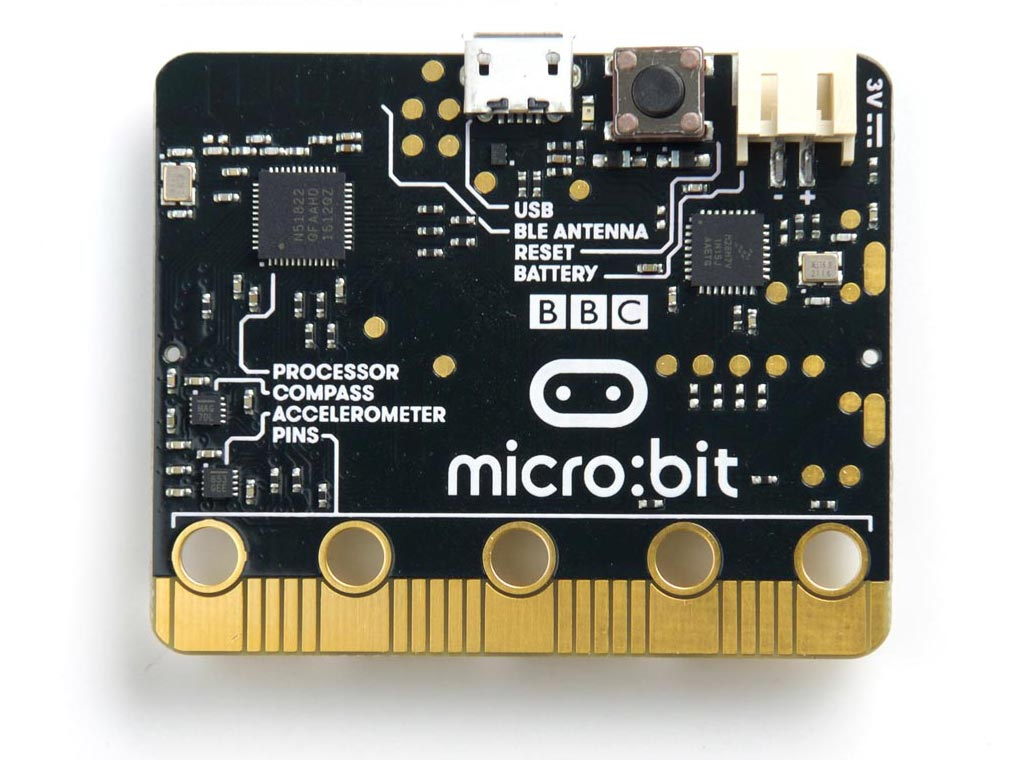#### Pin connections

The BBC Micro:bit provides 19 digital input/output pins, 6 analogue inputs, and 19 analogue outputs. They are accessible from the edge connector as follows: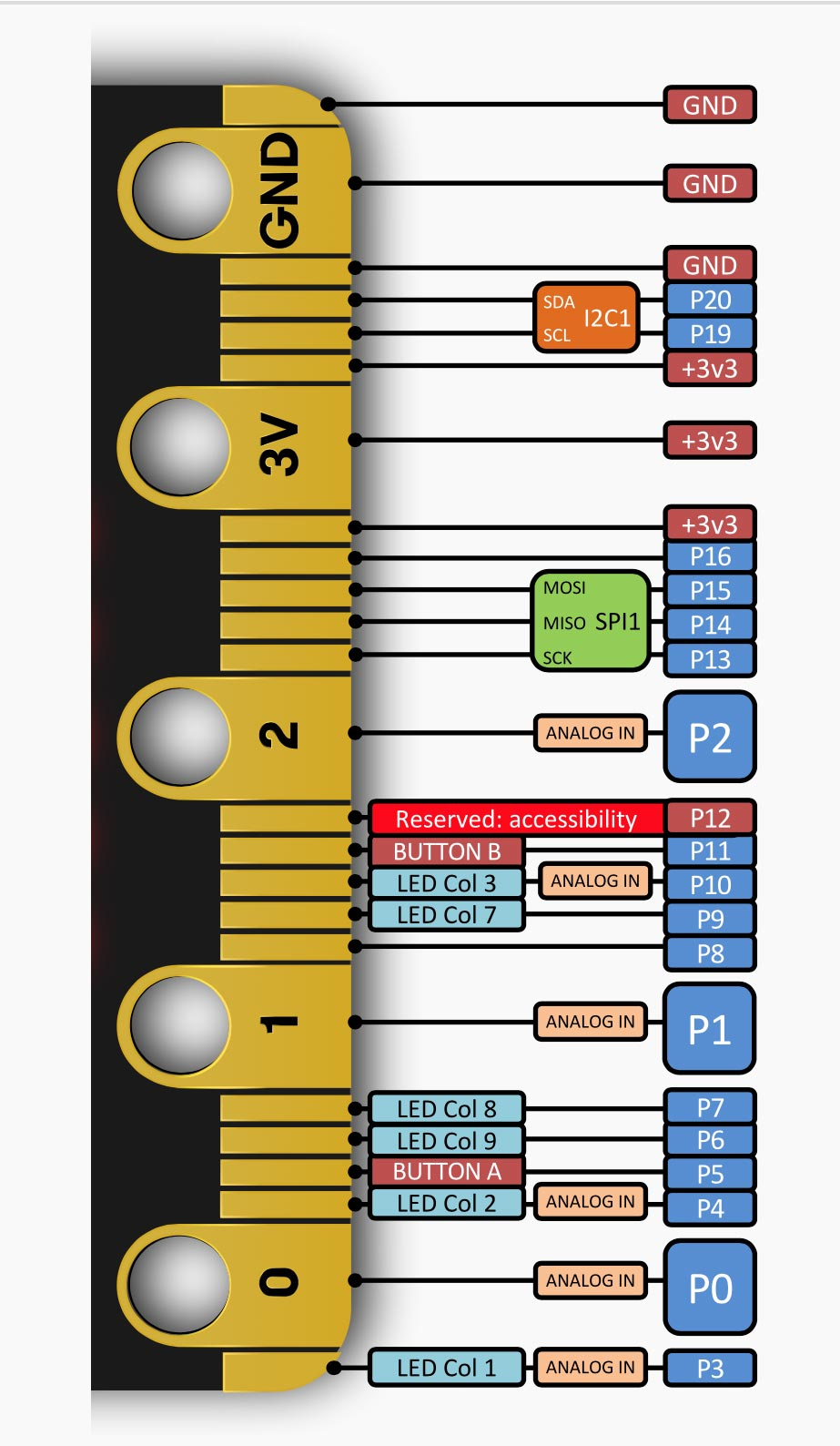The board also includes two pushbuttons and a matrix of 5 x 5 multiplexed LEDs. See Example programs below for examples of using these from uLisp.

#### Buttons

The reverse side of the BBC Micro:bit provides two pushbuttons on pins 5 and 11. You can read these with the following program:

```(defun buttons ()
(pinmode 5 :input)
(pinmode 11 :input)
(loop
(print
(delay 1000)))```

Run it by typing:

`(buttons)`

#### LEDs

The reverse of the BBC Micro:bit also provides a matrix of 5 x 5 LEDs. They are connected in a totally illogical arrangement of nine columns and three rows, and to light any individual LED you need to take the column low and the row high corresponding to that LED.

The following table shows the (row, column) for each LED:

 (26, 3) (27, 23) (26, 4) (27, 24) (26, 10) (28, 23) (28, 24) (28, 25) (28, 9) (28, 7) (27, 4) (26, 6) (27, 10) (28, 6) (27, 3) (26, 7) (26, 9) (26, 25) (26, 24) (26, 23) (28, 10) (27, 9) (28, 3) (27, 25) (28, 4)

This program flashes the LED at (row, column):

```(defun blink (row column)
(let (x)
(pinmode row :output)
(pinmode column :output)    (digitalwrite row t)
(loop
(digitalwrite column x)
(delay 1000)
(setq x (not x)))))```

For example, to flash the centre LED run:

`(blink 27 10)`

#### Analogue outputs

Any pin can also be used as an 8-bit analogue output, so you can pulsate the centre LED slowly on and off with the program:

```(defun pulse (row column)
(let (down)
(pinmode row :output)
(digitalwrite row t)
(loop
(dotimes (x 256)
(delay 5)
(analogwrite column (if down (- 255 x) x)))
(setq down (not down)))))```

Run it by typing:

`(pulse 27 10)`

Exit from any program by entering ~.

#### Analogue inputs

The BBC Micro:bit provides six 10-bit analogue inputs, three of which are available on the edge connector pins labelled 0, 1, and 2. The following example program reads the analogue input on pin 0 and displays the value as a bar of LEDs, using the fourth row of LEDs on the matrix: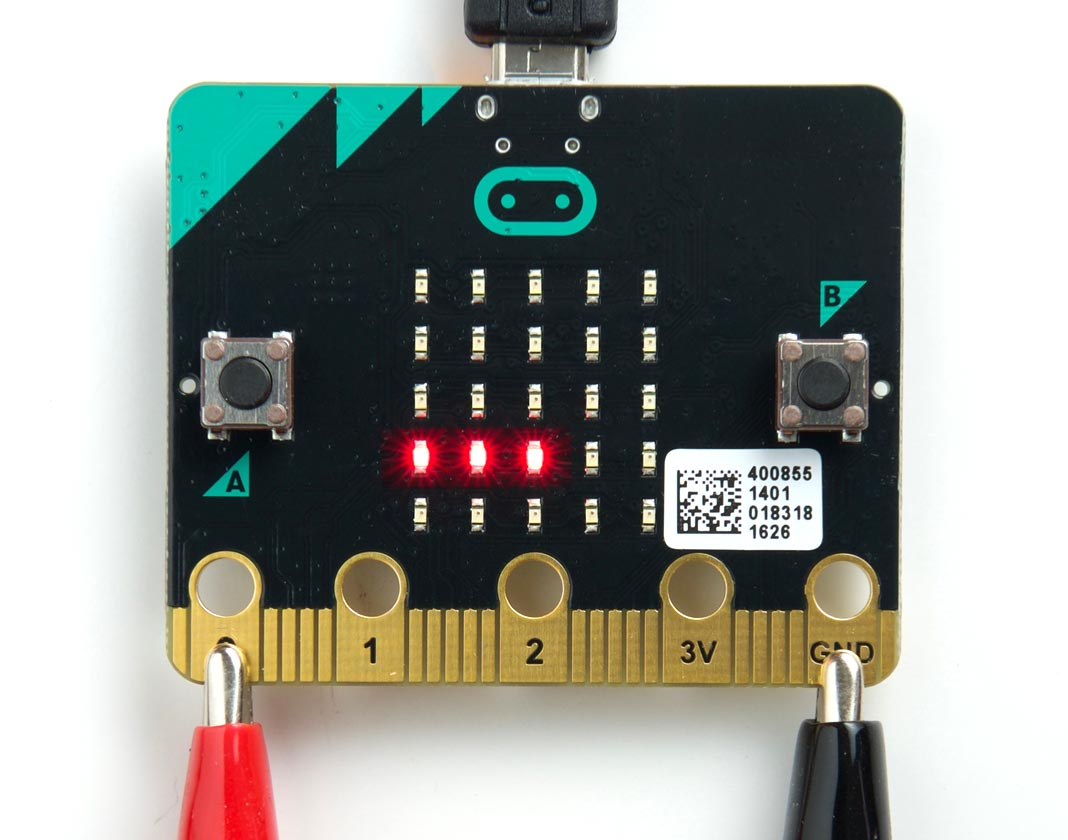The program takes advantage of the fact that this row of LEDs share the same row number. Here's the program:

```(defun meter ()
(let ((col '(7 9 25 24 23))
(row 26))
(pinmode row :output)
(digitalwrite row t)
(mapc (lambda (x) (pinmode x :output)) col)
(loop
(dotimes (n 5)
(digitalwrite
(nth n col)
(<
(* (1+ n) 170)))))))```

To run it evaluate:

`(meter)`

If you touch pin 0 on the edge connector you'll see the bar flash from the voltage on your finger. Connect a voltage of up to 3.3V to pin 0 to display a bar of LEDs in proportion to the voltage.

#### MMA8653FC 3-axis, 10-bit digital accelerometer

The Micro:bit includes an MMA8653FC accelerometer is on I2C address 29 (#x1D).

#### MAG3110 3-axis magnetometer

The Micro:bit includes an MAG3110 magnetometer is on I2C address 14 (0x0E). For details of using it see:

MAG3110 3-Axis Magnetometer

#### LSM303AGR accelerometer/magnetometer

Newer versions of the Micro:bit have a single LSM303AGR sensor which contains both an accelerometer and a magnetometer. For details of using it see:

LSM303D Accelerometer Magnetometer

1. ^ Edge Connector and Pinout on microbit.org.